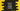# Python string isdigit() method explanation with example### Python string isdigit() method explanation with example:

The isdigit() method is an inbuilt method of Python string. It returns one boolean value based on all characters of the string are digit or not. It returns True if all characters are digits, else it returns False.

All characters with property value Numeric_Type=Digit or Numeric_Type=Decimal are called digits. This will also cover all digits which are not base-10 numbers.

In this post, we will learn how to use the isdigit() method with examples.

### Definition of isdigit:

The isdigit method is defined as like below:

``str.isdigit()``

Here, str is the string and isdigit() is called on this string str.

### Return value of isdigit:

The isdigit() method returns one Boolean value. It is True if all characters are digits, else False.

### Example of isdigit:

Let’s take an example of isdigit:

``````str_list = ['123', '0', '12.34', '+12', '-12', '012', '@12', 'abc123']

for s in str_list:
print(f'{s} => {s.isdigit()}')``````

In this program, str_list is a list of strings. It uses a for loop to iterate over these strings and prints the return value of isdigit() of each string.

If you run this program, it will print the below output:

``````123 => True
0 => True
12.34 => False
+12 => False
-12 => False
012 => True
@12 => False
abc123 => False``````

### Example of isdigit with superscript, subscripts and other numbers:

This method works with superscript, subscripts and other digits which have Numeric_Type=Digit or Numeric_Type=Decimal.

``````str_list = ['\u0035', '\u0036', '\u0037', '٦', '੭', '\u00B34']

for s in str_list:
print(f'{s} => {s.isdigit()}')``````

It will print True for all.